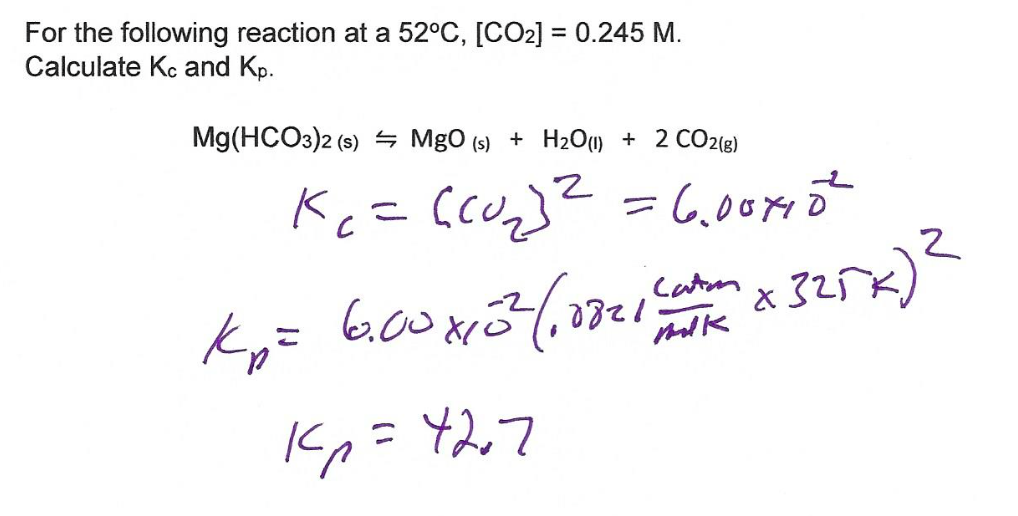# Question & Answer: I do not understand this solution given by my professor, could someone please explain why Kc…..I do not understand this solution given by my professor, could someone please explain why Kc does not include concentrations of product or the other reactants? thank you.

Don't use plagiarized sources. Get Your Custom Essay on
Question & Answer: I do not understand this solution given by my professor, could someone please explain why Kc…..
GET AN ESSAY WRITTEN FOR YOU FROM AS LOW AS \$13/PAGE

For the following reaction at a 52°C, [C02] = 0.245 M. Calculate Kc and Kp. Mg(HC03)2 (s) 늑 MgO(s) H2O(l) 2 CO2(g) + + 2 C.

First, let us define the equilibrium constant for any species:

The equilibrium constant will relate product and reactants distribution. It is similar to a ratio

The equilibrium is given by

rReactants -> pProducts

Keq = [products]^p / [reactants]^r

For a specific case:

aA + bB = cC + dD

Keq = [C]^c * [D]^d / ([A]^a * [B]^b)

Where Keq is constant at a given temperature, i.e. it is dependant on Temperature only

If Keq > 1, this favours products, since this relates to a higher amount of C + D

If Keq < 1, this favours reactants, since this relates to a higher amount of A + B

If Keq = 1, this is in equilibrium, therefore, none is favoured, both are in similar ratios

Note that the concentrations MUST be in equilibrium. If these are not in equilibrium, then the reaction will take place until there is equilibrium achieved.

Special notes:

Typically; we use aqueous and gas phases. (Gases and Aqueous Concentrations can be related via PV = nRT, since M = n/V as well)

Solids and Liquids, i..e. (s) and (l) have activity of 1. Therefore, they must not be considered in the ratios.

Then

Kc = [MgO] * [H2O] * CO2]^2 / [Mg(HCO3)2]

must be reduced to

Kc = [CO2]^2

and we know that

[CO2] = 0.245 M

so

Kc = (0.245)^2 = 0.060025 = 6*10^-2 approx

now,

relate Kc and Kp

Kp = Kc*(RT)^dn

R= 0.082 ideal gas constant, T = 52 C= ,325K dn = mol of gas changes, in this case, 2 mol of gas produced, so dn = 2

substitute

Kp =(0.060025)(0.082*325)^2

Kp = 42.63